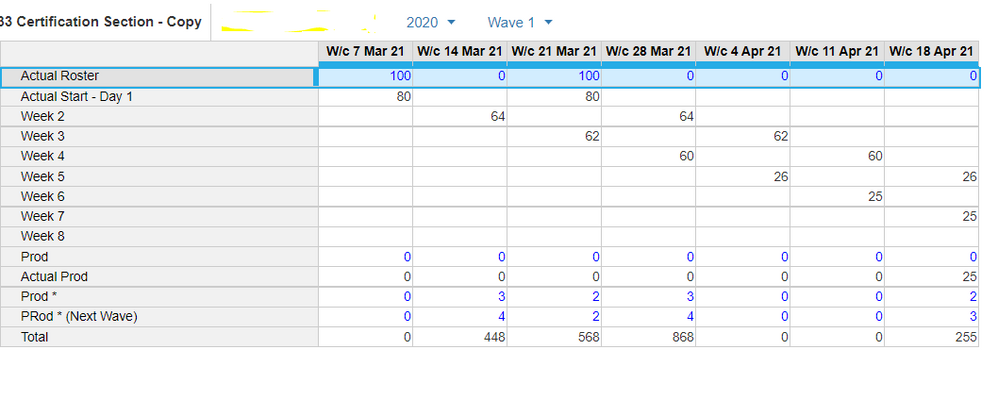# Multiplication and adding two different line itemsClass 1 is starting from 7 mar and class 2 is from 21 mar
Now i need to write formula in Total
For example in  21 mar Column, 62 * 2 (which is week 3 * prod )  and 80 * 2 (which is Actual Start - Day 1 * Prod  (Next Wave))
(62*2)+(80*2) = 284 ----- the formula in Total is not correct now

I try averaging the Prod and Prod Next Wave but the values can be like 8,3 so averaging not giving accurate calculations

Tagged: